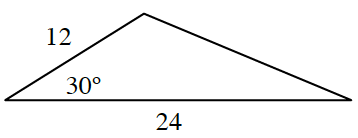### Home > CCG > Chapter 5 > Lesson 5.3.2 > Problem5-91

5-91.Find the area of the triangle at right. Show all work.

How can you divide this triangle so that you can find the height? Place the height in your diagram.

By drawing the height, a $30^\circ\text{-}60^\circ\text{-}90^\circ$ triangle with a hypotenuse of $12$ is created. What is the height?